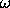## 5.12  Summary

Canonical transformations can be used to reformulate a problem in coordinates that are easier to understand or that expose some symmetry of a problem.

In this chapter we have investigated different representations of a dynamical system. We have found that different representations will be equivalent if the coordinate-momentum part of the transformation has a symplectic derivative, and if the Hamiltonian transforms in a specified way. If the phase-space transformation is time independent, then the Hamiltonian transforms by composition with the phase-space transformation. The symplectic condition can be equivalently expressed in terms of the fundamental Poisson brackets. The Poisson bracket and thefunction are invariant under canonical transformations. The invariance ofimplies that the sum of the areas of the projections onto fundamental coordinate-momentum planes is preserved (Poincaré integral invariant) by canonical transformations.

We can formulate an extended phase space in which time is treated as another coordinate. Time-dependent transformations are simple in the extended phase space. In the extended phase space the Poincaré integral invariant is the Poincaré-Cartan integral invariant. We can also reformulate a time-independent problem as a time-dependent problem with fewer degrees of freedom, with one of the original coordinates taking on the role of time; this is the reduced phase space.

A generating function is a real-valued function of the phase-space coordinates and time that represents a canonical transformation through its partial derivatives. We found that every canonical transformation can be represented by a generating function. The proof depends on the Poincaré integral invariant (and not on the fact that total time derivatives can be added to Lagrangians without changing the equations of motion).

The time evolution of any Hamiltonian system induces a canonical transformation: if we consider all possible initial states of a Hamiltonian system and follow all of the trajectories for the same time interval, then the map from the initial state to the final state of each trajectory is a canonical transformation. This is true for any interval we choose, so time evolution generates a continuous family of canonical transformations.

We generalized this idea to generate continuous canonical transformations other than those generated by time evolution. Such transformations will be especially useful in support of perturbation theory.

In rare cases a canonical transformation can be made to a representation in which the problem is easily solvable: when all coordinates are cyclic and all the momenta are conserved. Here we investigated the Hamilton-Jacobi method for finding such canonical transformations. For problems for which the Hamilton-Jacobi method works, we find that the time evolution of the system is given as a canonical transformation.